# Basic Proportionality Theorem(BPT)

Go back to  'Similar-Triangles'

Before we proceed with our discussion on similar triangles, it is important to understand a very fundamental theorem related to triangles: the basic proportionality theorem or BPT Theorem. This theorem is a key to understanding the concept of similarity better.

Basic Proportionality Theorem: If a line is drawn parallel to one side of a triangle to intersect the other two sides in distinct points, the other two sides are divided in the same ratio.

In the following figure, segment DE is parallel to the side BC of $$\Delta ABC$$. Note how DE divides AB and AC in the same ratio: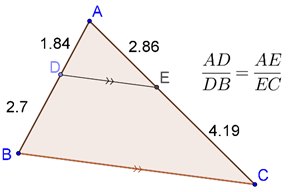The proof of this result is straightforward. Join BE and CD. Also, drop the perpendicular DP from D to AC, and the perpendicular EQ from E to AB, as shown below: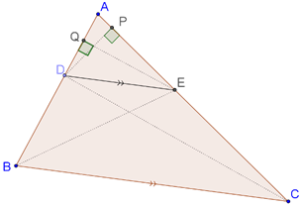Consider $$\Delta AED$$. If you have to calculate the area of this triangle, you can take AD to be the base, and EQ to be the altitude, so that:

${\rm{ar}}\left( {\Delta AED} \right) = \frac{1}{2} \times AD \times EQ$

Now, consider $$\Delta DEB$$. To calculate the area of this triangle, you can take DB to be the base, and EQ (again) to be the altitude. Thus:

${\rm{ar}}\left( {\Delta DEB} \right) = \frac{1}{2} \times DB \times EQ$

Next, consider the ratio of these two areas you have calculated:

$\frac{{{\rm{ar}}\left( {\Delta AED} \right)}}{{{\rm{ar}}\left( {\Delta DEB} \right)}} = \frac{{\frac{1}{2} \times AD \times EQ}}{{\frac{1}{2} \times DB \times EQ}} = \frac{{AD}}{{DB}}$

In an exactly analogous manner, you can evaluate the ratio of areas of $$\Delta AED$$ and $$\Delta EDC$$:

$\frac{{{\rm{ar}}\left( {\Delta AED} \right)}}{{{\rm{ar}}\left( {\Delta EDC} \right)}} = \frac{{\frac{1}{2} \times AE \times DP}}{{\frac{1}{2} \times EC \times DP}} = \frac{{AE}}{{EC}}$

Finally, note that the areas of $$\Delta DEB$$ and $$\Delta EDC$$ must be the same, since they are on the same base DE, and between the same parallels – DE and BC. Thus,

\begin{align}&\frac{{{\rm{ar}}\left( {\Delta AED} \right)}}{{{\rm{ar}}\left( {\Delta DEB} \right)}} = \frac{{{\rm{ar}}\left( {\Delta AED} \right)}}{{{\rm{ar}}\left( {\Delta EDC} \right)}}\\& \Rightarrow \;\;\;\frac{{AD}}{{DB}} = \frac{{AE}}{{EC}}\end{align}

This completes our proof of the fact that DE divides AB and AC in the same ratio. Note that the fact that DE is parallel to BC was crucial to the proof. Without this, the areas of $$\Delta DEB$$ and $$\Delta EDC$$ would not have been the same, and hence the two ratios would have been different.

Can we say that the converse of the Basic Proportionality Theorem (BPT) will hold? That is, if in a triangle, a line segment divides two sides in the same ratio, will it be parallel to the third side? The answer is: yes. Let’s prove this result.

## Converse of the BPT

In a triangle, if a line segment intersecting two sides divides them in the same ratio, then it will be parallel to the third side.

## Proof of BPT

Consider the following figure, and let it be given that AD:DB = AE:EC. Suppose that DE is not parallel to BC. Draw segment DF through D which is parallel to BC, as shown: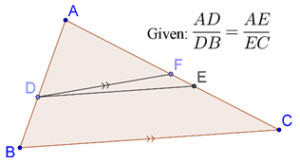Using the BPT, we see that DF should divide AB and AC in the same ratio. Thus, we should have:

$\frac{{AD}}{{DB}} = \frac{{AF}}{{FC}}$

But it is already given to us that:

$\frac{{AD}}{{DB}} = \frac{{AE}}{{EC}}$

This means that:

\begin{align}&\frac{{AE}}{{EC}} = \frac{{AF}}{{FC}}\\ &\Rightarrow \quad \frac{{AE}}{{EC}} + 1 = \frac{{AF}}{{FC}} + 1\\& \Rightarrow \quad \frac{{AE + EC}}{{EC}} = \frac{{AF + FC}}{{FC}}\\ &\Rightarrow \quad \frac{{AC}}{{EC}} = \frac{{AC}}{{FC}}\\&\Rightarrow \quad EC = FC\end{align}

This cannot happen if E and F are different points. Thus, we can conclude that DE is parallel to BC, hence completing the proof of the converse of the BPT.

## Solved Examples

Example 1: Consider $$\Delta ABC$$, in which segment $$DE$$ parallel to BC is drawn from D on AB to E on BC, as shown below: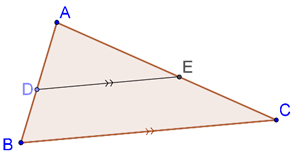Show that $$\Delta ADE$$ is similar to $$\Delta ABC$$.

Solution: Recall that two triangles are said to be similar if they should be equi-angular (that is, corresponding angles are equal), and corresponding sides are be proportional. It should be obvious that $$\Delta ADE$$ and $$\Delta ABC$$ are equi-angular:

1. $$\angle A$$ = $$\angle A$$ (common angle)

2. $$\angle ADE$$ = $$\angle ABC$$ (corresponding angles)

3. $$\angle AED$$ = $$\angle ACB$$ (corresponding angles)

Now, we will show that the corresponding sides are proportional. Using the BPT, we have:

\begin{align}&\frac{{AD}}{{DB}} = \frac{{AE}}{{EC}}\\ &\Rightarrow \quad \frac{{DB}}{{AD}} = \frac{{EC}}{{AE}}\\& \Rightarrow \quad \frac{{DB}}{{AD}} + 1 = \frac{{EC}}{{AE}} + 1\\& \Rightarrow \quad \frac{{DB + AD}}{{AD}} = \frac{{EC + AE}}{{AE}}\\& \Rightarrow \quad \frac{{AB}}{{AD}} = \frac{{AC}}{{AE}}\\& \Rightarrow \quad \frac{{AD}}{{AB}} = \frac{{AE}}{{AC}}\end{align}

We have thus shown two pairs of sides to be proportional. All that remains to be shown that the ratios above are also equal to the ratio of the third pair, that is, to DE:BC. To prove that, draw segment EF parallel to AB, as shown: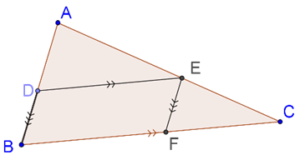Since EF || AB, the BPT tells us that:

\begin{align}&\frac{{CE}}{{EA}} = \frac{{CF}}{{FB}}\\ &\Rightarrow \quad \frac{{CE}}{{EA}} + 1 = \frac{{CF}}{{FB}} + 1\\ &\Rightarrow \quad \frac{{CE + EA}}{{EA}} = \frac{{CF + FB}}{{FB}}\\ &\Rightarrow \quad \frac{{AC}}{{AE}} = \frac{{BC}}{{FB}}\end{align}

But BF is equal to DE (why?), and so:

\begin{align}&\frac{{AC}}{{AE}} = \frac{{BC}}{{DE}}\\ &\Rightarrow \quad \frac{{AE}}{{AC}} = \frac{{DE}}{{BC}}\end{align}

Thus, we finally have:

$\frac{{AD}}{{DB}} = \frac{{AE}}{{AC}} = \frac{{DE}}{{BC}}$

This proves that all the three pairs of sides are proportional, which means that the two triangles are similar.

The result we have discussed here is extremely important and will be used frequently, so it is imperative that you go through the discussion again if you have not followed it completely.

Example 2: ABCD is a trapezium such that AB || DC. E and F are points on non-parallel sides AD and BC respectively such that EF || AB, as shown below: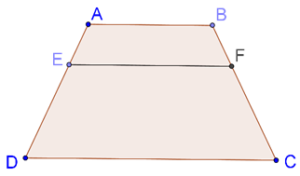Show that AE:ED = BF:FC

Solution: Join BD, and let it intersect EF at G, as shown below: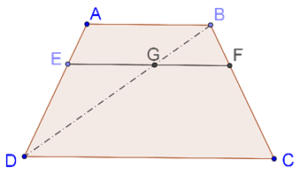Now, we will apply the BPT twice, once in $$\Delta ADB$$, and once in $$\Delta DBC$$. We have:

\left\{ \begin{align}&\frac{{AE}}{{ED}} = \frac{{BG}}{{GD}}\\&\frac{{BG}}{{GD}} = \frac{{BF}}{{FC}}\end{align} \right.

Clearly,

$\frac{{AE}}{{ED}} = \frac{{BF}}{{FC}}$

Example 3: Consider the following figure: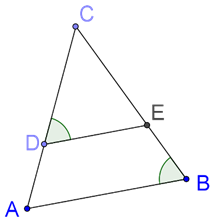It is given that CD:DA = CE:EB, and $$\angle CDE$$ = $$\angle CBA$$. Prove that $$\Delta CAB$$ is isosceles.

Solution: The fact that CD:DA = CE:EB tells us that DE || AB (by the converse of the BPT). Thus,

$$\angle CDE$$ = $$\angle CAB$$ (corresponding angles)

But it is given that $$\angle CDE$$ = $$\angle CBA$$, which means that

$$\angle CAB$$ = $$\angle CBA$$

This means that CA = CB, and hence $$\Delta CAB$$ is isosceles.

Learn math from the experts and clarify doubts instantly

• Instant doubt clearing (live one on one)
• Learn from India’s best math teachers
• Completely personalized curriculum